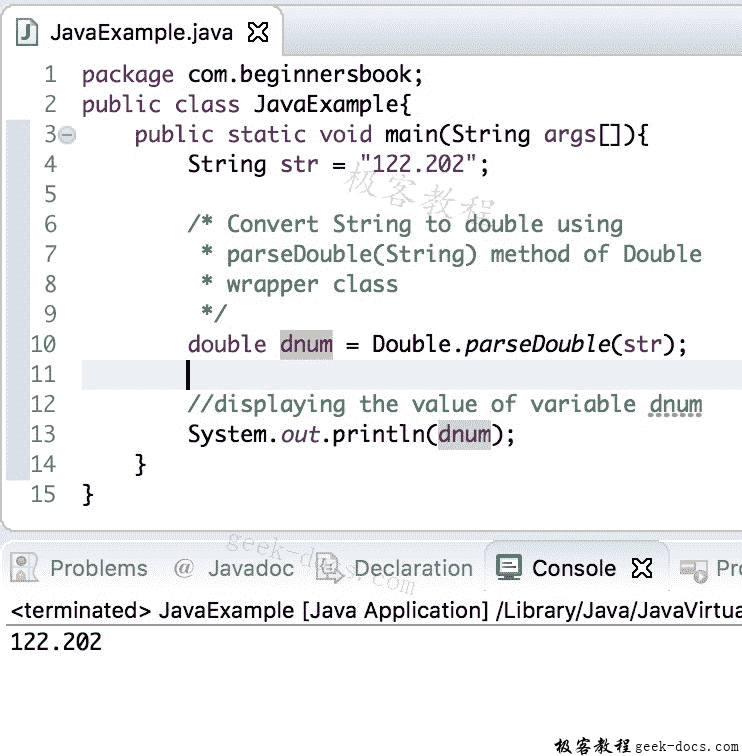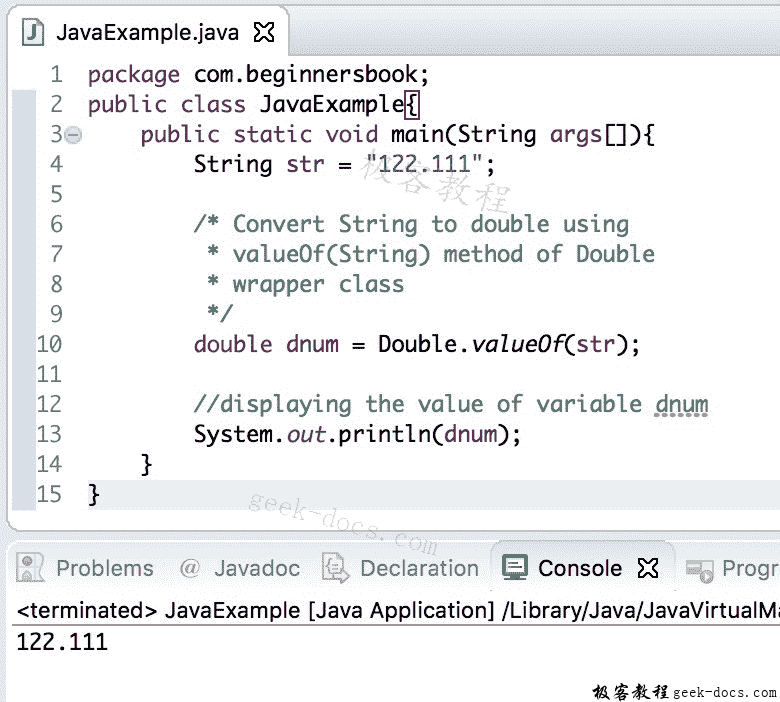# Java 实例 String到double的转换

1. 使用Double.parseDouble(String)方法将String转换为Double
2. 使用Double.valueOf(String)String转换为Double
3. 使用Double类的构造函数转换为Double自 Java 版本 9 以来，不推荐使用构造函数Double(String)

## 使用Double.parseDouble(String)转换为Double

public static double parseDouble(String str) throws NumberFormatException


String str="122.202";
double dnum = Double.parseDouble(str);


public class JavaExample{
public static void main(String args[]){
String str = "122.202";

/* Convert String to double using
* parseDouble(String) method of Double
* wrapper class
*/
double dnum = Double.parseDouble(str);

//displaying the value of variable dnum
System.out.println(dnum);
}
}## 使用Double.valueOf(String)转换为Double

Java 中Double包装类的valueOf()方法与我们在上面 java 示例中看到的parseDouble()方法类似。

String str = "122.111";
double dnum = Double.valueOf(str);


public class JavaExample{
public static void main(String args[]){
String str = "122.111";

/* Convert String to double using
* valueOf(String) method of Double
* wrapper class
*/
double dnum = Double.valueOf(str);

//displaying the value of variable dnum
System.out.println(dnum);
}
}## 使用Double类的构造函数将String转换为double

String str3 = "999.333";
double var3 = new Double(str3);


Double类有一个构造函数，它解析我们在构造函数中传递的String参数，并返回一个等效的double值。

public Double(String s) throws NumberFormatException


public class Example{
public static void main(String args[]){
String str3 ="999.333";
double var3 = new Double(str3);
System.out.println(var3);
}
}



999.333



• 回顶